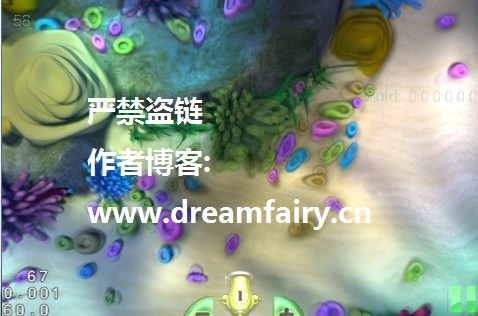## 在Stage3D中实现X-Ray透视效果1.渲染所有物体+场景
2.正常渲染主角

[cc lang=”actionscript3″]
public override function getFragmentProgram():ByteArray
{
var index : uint;
10/255,72/255,247/255,0.5,
1,0,0,0]);

var code : String =
“nrm ft1.xyz v”+vProjPos+”.xyzn” +
“dp3 ft0.a ft1.xyz fc”+fcCameraPos+”.xyzn”+
“sat ft0.a ft0.an”+
“mov ft0.r fc”+fcXray+”.rn”+
“mov ft0.g fc”+fcXray+”.gn”+
“mov ft0.b fc”+fcXray+”.bn”+
“mul ft0.rgb ft0.rgb ft0.aaan”+
“mov oc ft0n”;

return new AGALMiniAssembler().assemble(Context3DProgramType.FRAGMENT, code);
}
[/cc]

fcXray 的值为
[10/255,72/255,247/255,0.5,1,0,0,0]

fcCameraPos 为外部传入的相机位置，已经归一化和反转过

## 移植:在Cocos2d-X中实现水波滤镜

《cocos2d-x 权威指南》
《cocos2d-x 高级开发教程》## 使用四元数来渲染蒙皮动画

[cc lang=”actionscript3″]
var datas : Vector. = matrix3D.decompose(Orientation3D.QUATERNION);
[/cc]

datas 是一个数组，其实是位移，是四元数，是缩放

[cc lang=”actionscript3″]
var quaIndex : uint = index / 2;
var rotation : Vector3D = m_jointConstant[quaIndex];
var translate : Vector3D = m_jointConstant[quaIndex + 1];

var mat : Matrix3D = new Matrix3D();

var vt0 : Vector3D = new Vector3D();
vt0.x = 2 * rotation.x * rotation.y;
vt0.y = 2 * rotation.x * rotation.z;
vt0.z = 2 * rotation.x * rotation.w;
vt0.w = 2 * rotation.y * rotation.z;
var vt1 : Vector3D = new Vector3D();
vt1.x = 2 * rotation.y * rotation.w;
vt1.y = 2 * rotation.z * rotation.w;
vt1.z = rotation.x * rotation.x;
vt1.w = rotation.y * rotation.y;
var vt2 : Vector3D = new Vector3D();
vt2.x = rotation.z * rotation.z;
vt2.y = rotation.w * rotation.w;

var rawData : Vector. = mat.rawData;
xx – yy – zz + ww
rawData = vt1.z – vt1.w – vt2.x + vt2.y;
2xy – 2zw
rawData = vt0.x – vt1.y;
2xz + 2yw
rawData = vt0.y + vt1.x;
0
rawData = translate.x;
2xy + 2zw
rawData = vt0.x + vt1.y;
-xx + yy – zz + ww
rawData = vt1.w – vt1.z – vt2.x + vt2.y;
2yz + 2xw
rawData = vt0.w – vt0.z;
0
rawData = translate.y;
2xz + 2yw
rawData = vt0.y – vt1.x;
2yz – 2xw
rawData = vt0.w + vt0.z;
-xx – yy + zz + ww
rawData = vt2.x – vt1.z – vt1.w + vt2.y;
0
rawData = translate.z;
0
rawData = 0;
0
rawData = 0;
0
rawData = 0;
1
rawData = 1;

mat.rawData = rawData;
[/cc]